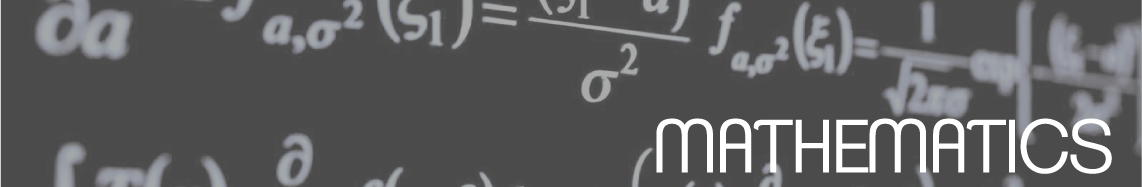We develop different models, algorithms and codes to face on the simulation of Heat and Mass Transfer Problems, the simulation of electromagnetic propagation in optical systems and on the optimization of problems in the field of Bio-Systems. We have a solid background in developing numerical methods based on finite difference or modal techniques and in developing Monte Carlo computer codes for simulating different complex physical problems.

#### Routing Optimization

Many real situations can be modeled as routing optimization problems. Usually it is possible to design exact algorithms to solve some examples but in most cases, its complexity makes necessary to resort to heuristic algorithms. Exact procedures are based on the polyhedral description of the set of possible solutions to apply Linear Programming techniques. Heuristic algorithms take advantage of the high numerical capacity of present computers to generate a great amount of possible solutions to find the optimal one. Different techniques exist to perform this search: Monte Carlo, genetic algorithms, Tabu Search, GRASP... Our group has experience in both types of approaches.

#### Computation of Special Functions

In many fields of Physics and Engineering is quite common the calculation of special functions, for instance Bessel functions(BF) of different orders. Our group has experience in the design of fast codes of computation for the evaluation of (high order) BF based on the continued fractions method. In fact, the usual methods for the BF evaluation incorporate renormalization relations. We have developed algorithms to evaluate BF, both regular and irregular, without using re-evaluation with the help of these relations. In addition, our methods maintain the stability of each recurrence relation, i.e. they use forward recurrence relations for the BF of second class and backward relations for the first class BF.

#### Numerical simulation on Heat and Mass Transfer Problems

In this topic, our group has devoted a lot of effort to develop numerical methods -based on techniques of finite differences, finite elements and finite volumes- for the simulation of conduction, convection and radiation problems.

#### Monte Carlo Methods

We have experience in developing Monte Carlo computer codes for simulating different complex physical problems (for instance, in Nuclear Physics), and also when solving different applied mathematic problems, like routing problems, multidimensional integration, partial differential equations, etc.

#### Numerical Methods in Photonics

We have developed software for simulating (linear and nonlinear) electromagnetic propagation in optical systems, mainly optical fibres, following discretization schemes (finite differences and finite elements techniques) as well as modal methods. Then we study the problem of finding and analysing soliton solutions in optical fibres.

#### Current trends in Mathematical Physics

Current trends in mathematical physics (especially those concerning the relation between gravity and quantum mechanics) demand a rethinking of some of the foundations of quantum theory. Physicists as renowned as Nobel Laureate G. ‘t Hooft call for such a rethinking. The final goal is to improve our understanding of quantum gravity and to shed light on some of its least understood facets. The latter include: the information loss paradox, the cosmological constant, and dark energy and dark matter.

In order to tackle these difficulties it has been suggested that quantum mechanics might “emerge”, as a statistical theory, from an underlying deterministic theory, after a “dissipation” or “thermalisation” process. Following this approach it has been established that, given an arbitrary quantum system, there exists an underlying deterministic mechanics which, after “thermalisation”, reproduces the given quantum dynamics one started out with.

In recent publications our group has found that the above named “dissipation”, or “thermalisation”, can be modelled on the basis of the classical thermodynamics of irreversible processes as developed by Nobel Laureates L. Onsager and I. Prigogine.

Universitat Politècnica de València - Universitat de València